# Net force of object indicated in following diagram

• Evangeline101
In summary, the homework statement is to calculate the net force acting on an object indicated in the diagrams.

## Homework Statement

Calculate the net force acting on the object indicated in the following diagrams. Show your work.

1)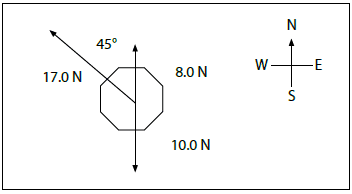2)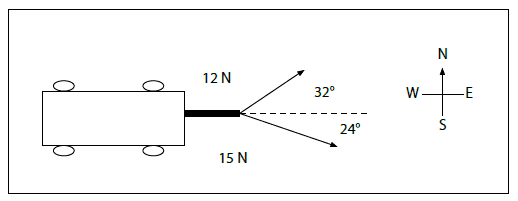## Homework Equations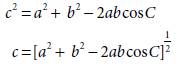[/B]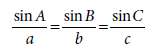## The Attempt at a Solution

1)
8.0 N (north) + 10.0 N (south)
- 8.0 N (south) + 10.0 N (south)
= 2.0 N (south)

c= [ (2.0 N) 2 + (17.0 N) 2 - 2 (2.0 N) x (17.0 N) cos 45° ] 1/2
c= 15.6 N

sinA/2.0N = sin45°/15.6N

A= 5.2°
A= 45° - 5.2° = 39.8°

Fnet = 15.6 N [N 39.8° W]

2)
c= [ (12 N) 2 + (15 N)2 - 2 (12 N) x (15 N) cos124°]
c= 23.9 N

sinA/15N = sin124°/ 23.9 N

A = 31°

Fnet = 23.9 N [ N 31° E]

is this correct?

I agree with both magnitudes.

In 1, you do not show a diagram for the triangle of forces, so I'm not sure what your angle A is. You seem to have used it inconsistently, leading to the wrong answer for direction of force.

In 2, the angle you found is not the one you want. It seems to be the angle between the resultant and the 12N force.

Can you post your force triangle diagrams?

haruspex said:
In 1, you do not show a diagram for the triangle of forces, so I'm not sure what your angle A is. You seem to have used it inconsistently, leading to the wrong answer for direction of force.

1)
Here is the diagram for 1) :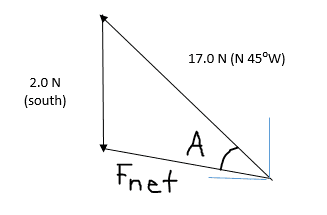I corrected my answer for 1) :

8.0 N (north) + 10.0 N (south)

-8.0 N (south) + 10.0 N (south)

= 2.0 N (south)

c = [(2.0 N)2 + (17.0 N)2- 2 (2.0 N) x (17.0 N) cos 45°]1/2

c = 15.6 N

sinA/a = sinB/b = sinC/c

sinA/2.0 N = sin 45°/15.6 N

A = 5.2°

45° - 5.2° = 39.8°

Fnet = 16 N [ W 40° N]

is this better?

Last edited:
haruspex said:
In 2, the angle you found is not the one you want. It seems to be the angle between the resultant and the 12N force.

2)
Here is the diagram for 2):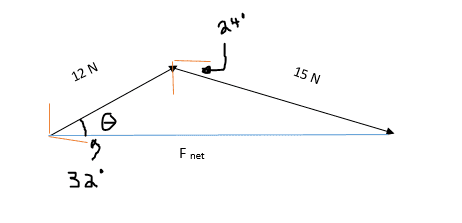I also corrected my answer for 2) :

c2 = a2 + b2 - 2abcosC

c = (a2 + b2 - 2abcosC)1/2

c = [(12 N)2 + (15 N)2- 2 (12 N) x (15 N) cos 124°]1/2

c = 23.9 N

sinA/a = sinB/b = sinC/c

sinA/15 N = sin 124°/ 23.9 N

A = 31°

32° - 31° = 1°

90° - 1° = 89°

Fnet = 24.0 N [N 89° E] or 24.0 N [E 1° N]

better?

Last edited:
Evangeline101 said:
1)
Here is the diagram for 1) :

View attachment 100369
I corrected my answer for 1) :

8.0 N (north) + 10.0 N (south)

-8.0 N (south) + 10.0 N (south)

= 2.0 N (south)

c = [(2.0 N)2 + (17.0 N)2- 2 (2.0 N) x (17.0 N) cos 45°]1/2

c = 15.6 N

sinA/a = sinB/b = sinC/c

sinA/2.0 N = sin 45°/15.6 N

A = 5.2°

45° - 5.2° = 39.8°

Fnet = 16 N [ W 40° N]

is this better?
Looks right.

Edit: the angle might be a bit inaccurate. Retry it keeping more significant digits at each step.
Doing it a slightly different way (using arctan) I got about 42 degrees.

Last edited:
Evangeline101 said:
2)
Here is the diagram for 2):
View attachment 100371

I also corrected my answer for 2) :

c2 = a2 + b2 - 2abcosC

c = (a2 + b2 - 2abcosC)1/2

c = [(12 N)2 + (15 N)2- 2 (12 N) x (15 N) cos 124°]1/2

c = 23.9 N

sinA/a = sinB/b = sinC/c

sinA/15 N = sin 124°/ 23.9 N

A = 31°

32° - 31° = 1°

90° - 1° = 89°

Fnet = 24.0 N [N 89° E] or 24.0 N [E 1° N]

better?
yes, but again the angle could be a touch more accurate.

haruspex said:
Edit: the angle might be a bit inaccurate. Retry it keeping more significant digits at each step.
Doing it a slightly different way (using arctan) I got about 42 degrees.

Ok, I will keep a few more significant digits to make the angle more accurate. Anyway, thanks for the help :)